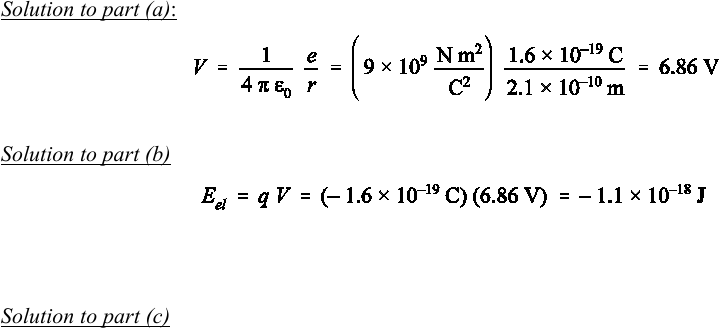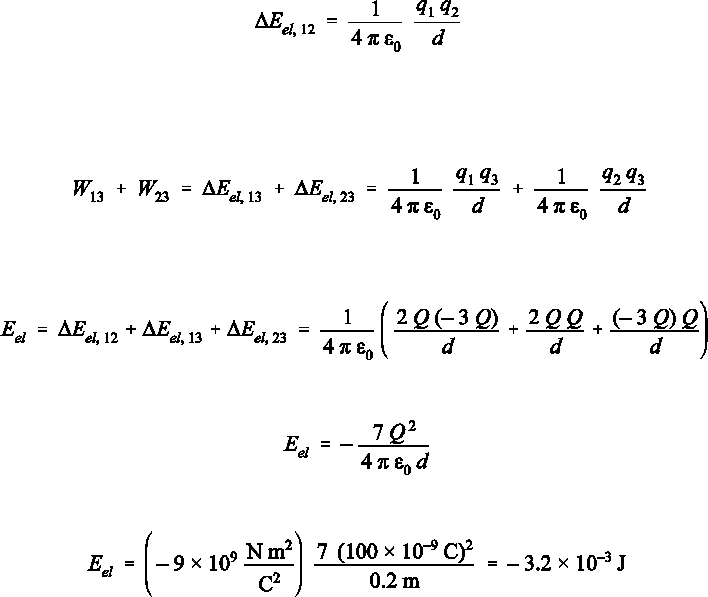# Physics 1028 Chapter Notes - Chapter 18: Centripetal Force, Electric Potential Energy, Electric Potential

2 views6 pages
School
Western University
Department
Physics
Course
Physics 1028
ProfessorSelection of Even–Numbered Problem Solutions
Chapter 18
P–18.2
Solution to part (a): Eq. [18.8] gives the potential for a point charge:
(1)
Solution to part (b): The electric potential energy follows from the charge and the potential:
(2)
in which the negative sign is due to the opposite signs of the two interacting charges. This means that the
electron is attracted by the proton.
Solution to part (c): As the electron comes closer to the proton the electric potential energy becomes even
more negative than calculated in Eq. . Since the electron and proton attract each other, this is the same
situation as we saw before for gravity, where two masses always attract each other and the gravitational
potential energy is lowered when the masses approach each other (e.g., when objects fall toward the surface
of Earth). In a classical sense, the electron does not crash into the proton for the same reason the Moon
doesn’t fall down onto Earth: the circular motion (Moon around Earth or electron around proton) leads to an
apparent centrifugal effect balancing the attractive force (phrased proper, the attractive force will be the
centripetal force for a circular motion)..
Unlock document

This preview shows pages 1-2 of the document.
Unlock all 6 pages and 3 million more documents.P–18.4
Following the hint, we built the system of charges by moving one after the other from a place where they are
all at infinite distance to each other. Since the electric force decreases rapidly with distance, a distance
equivalent to an infinite distance might practically be a rather short distance. In vacuum this distance is quite
large for a precise measurement; however, in a solution a few micrometres is an infinite distance due to
charge screening effects. The electric energy of the system is Eel = 0 J while the charges are at infinite
distance to each other, i.e., while they do not interact with each other.
When we bring q1 to its final position, the electric energy remains zero, i.e., Eel = 0 J, since the charge
still does not interact with any other charge. Next we bring q2 to its final position, which is at a distance d
from charge q1. In this process the change in the electric potential energy is:
(3)
In the last step we bring q3 to its final position at the distance d to either of the other two point
charges. The work associated with this step is equal to the sum of the work to bring q3 to a distance d from
q1 and the work to bring q3 to a distance d from q2:
(4)
The total change of the electric potential energy is the sum of the contributions due to each of the three steps
toward the final charge arrangement:
(5)
This yields:
(6)
substituting the given values leads to the final result:
(7)
Since the work calculated in this equation is negative, we know that the source of an external force has to do
this amount of work to disassemble the structure of charges as shown in Fig. 18.31. In turn, creating the
arrangement of charges shown in Fig. 18.31 by starting with the three charges infinitely separated releases
3.2 mJ of energy.
Unlock document

This preview shows pages 1-2 of the document.
Unlock all 6 pages and 3 million more documents.

## Document Summary

[18. 8] gives the potential for a point charge: Solution to part (b): the electric potential energy follows from the charge and the potential: (1) (2) in which the negative sign is due to the opposite signs of the two interacting charges. This means that the electron is attracted by the proton. Solution to part (c): as the electron comes closer to the proton the electric potential energy becomes even more negative than calculated in eq. Following the hint, we built the system of charges by moving one after the other from a place where they are all at infinite distance to each other. Since the electric force decreases rapidly with distance, a distance equivalent to an infinite distance might practically be a rather short distance. In vacuum this distance is quite large for a precise measurement; however, in a solution a few micrometres is an infinite distance due to charge screening effects.